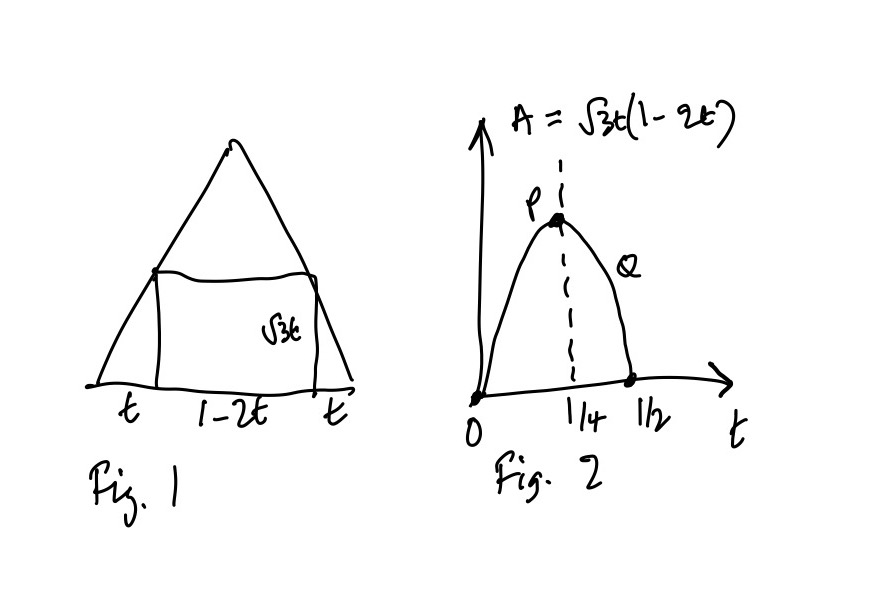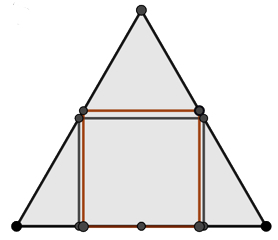## Solution to square in a triangle problem

Visible to anyone in the world
Edited by Richard Walker, Sunday, 19 Jun 2022, 14:05

This is a solution to the question I asked on 13 June, what is the rectangle of largest area that can be inscribed in an equilateral triangle of side 1?

Intuitively we might guess that the rectangle concerned is a square but it turns out that it isn't.Suppose the. base of the rectangle is as shown in Fig. 1. Then we can use trigonometry to show its height is sqrt(3)t and find its area. Fig. 2 shows a graph of the area against t, which is a parabola with a line of symmetry as show, and a vertex P which corresponds to the maximum area. The graph must pass through the point where t = 0, so the width of the rectangle is 1 and its height and therefore area is 0. It must also pass through the point where t = ½ and the width of the rectangle is 0 and therefore its area is again 0. By symmetry the vertex of the parabola must be half-way between these values of t, so the maximum is when t = ¼.

This rectangle has an area which is exactly 50% of the are of the triangle. It's interesting to compare this with the area of the largest square, which is very close, at about 49.7% of the area of the triangle. I drew the diagram below in GeoGebra showing the rectangle in black and the square in brown. You can see how small the difference is.Share post

This blog might contain posts that are only visible to logged-in users, or where only logged-in users can comment. If you have an account on the system, please log in for full access.

Total visits to this blog: 1598273